# Vector Addition – Analytical Method

## Notes

Adding two or more vectors is called vector addition. What is a vector? Any quantity which has magnitude along with direction is a vector. Some of the examples for vector quantities are displacement, velocity, acceleration, force, pressure etc. Mathematical operations can be performed between two or more vectors. In this article, we will learn about the vector addition of two quantities using the analytical methods.

The process of adding two or more vectors is called vector addition. Depending on the direction of the vector, vector addition is categorised into two types. They are –

Consider a vector bar A and bar B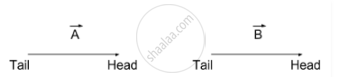If two vectors are arranged head to head or tail to tail then, the parallelogram law of vector addition is carried out.

Statement
“If two vectors are considered to be the adjacent sides of a parallelogram, then the resultant of two vectors is given by the vector that is a diagonal passing through the point of contact of two vectors.”

Method
Step-wise vector addition of two vectors using Parallelogram law of vector addition is given below-
Step 1: Consider two vectors; bar A and bar B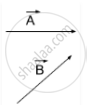Step 2: Bring the tail of bar A to the tail of bar B. Here the direction of vectors is not changed.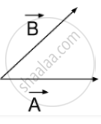Step 3: Draw a lines parallel to bar A and bar B with the same magnitude, in a way to complete parallelogram.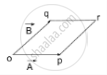Step 4: Join the point o and r by a straight line with an arrow pointing towards the r. This is diagonal to the parallelogram.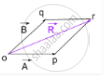And this is the resultant vector bar R, bar A + bar B = bar R

If two vectors are arranged head to tail the traingular law of vector addition is carried out.

Statement:
“When two vectors are represented by two sides of a triangle in magnitude and direction were taken in the same order then the third side of that triangle represents in magnitude and direction the resultant of the vectors.”

Method:
Step-wise vector addition of two vectors using Triangular law of vector addition is given below-
Step 1: Consider two vectors, bar A and bar B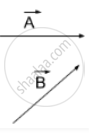Step 2: Bring the head of bar A to the tail of bar B. Here the direction of vectors is not changed.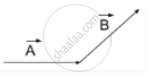Step 3: Join the tail of bar Ato the head of bar Bby a straight line with an arrow pointing towards the head of bar B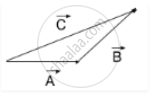This new vector is the resultant vector bar C, bar A + bar B = bar C